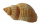# Division + addition - math problems

#### Number of problems found: 47

• Jose studiedJose studied for 4 and 1/2 hours on Saturday and another 6 and 1/4 hours on Sunday. How many subjects did he study if he has alloted 1 and 1/2 hours per subject on Saturday and 1 and 1/4 hours per subject on Sunday?
• Nice two numbersWhat two numbers we give a sum of 30 difference of 24 products of 81 and a quotient of 9?
• Simplify expression with mixedWhen (7¹/3 + 2¾)÷(2 + 2¼ × 1¹/3) is simplified the result is?
• Quotient and productIf the quotient of [8/5 divided by 8/10] is added to the product of [8/14 x 7/12 x 3/8], what is the sum?
• The cost 2The cost of 5 apples is \$3.45  and 5 oranges is \$1.23. If Rachel buys one apple and one orange, then how much must she pay?
• ItemsItems Acosts 675.35 while item B costs 1605.25. by how much more was the cost of item B than item A. If item A and B will be purchased, how much would are pay?
• Evaluate 11Multiply the quotient of 6 and 2 by 3, then add 1. Add 1 and 6, then divide by 2 and multiply by 3. Divide 6 by the product of 2 and 3, then add 1.
• Evaluate valueEvaluate value of the following expressions: 1. The product of nine and a number 12, decreased by six 2. Eight decreased by three times a number 2. 3. The quotient of twice a number 49 and seven.
• Fractions and mixed numerals(a) Convert the following mixed numbers to improper fractions. i. 3 5/8 ii. 7 7/6 (b) Convert the following improper fraction to a mixed number. i. 13/4 ii. 78/5 (c) Simplify these fractions to their lowest terms. i. 36/42 ii. 27/45 2. evaluate the follow
• AngelaAngela shared a cab with her friends. When they arrived at their destination, they evenly divided the \$120 fare among the 4 of them. Angela also paid a 5 dollar tip. How much did Angela pay in total?
• Fruit punchKelly made fruit punch to serve at a party for her chess team. She mixed 1 2/5  liters of cranberry juice and 1 3/5  liters of pineapple juice together. Then, she split the fruit punch evenly among nine glasses. How much fruit punch did Kelly pour into ea
• Painting classroomsMartha solicited 3 2/3 liters of paint for the brigade. Their city mayor gave their school another 2 2/5 liters of paint. If each classroom needs 1 3/7 liters, how many classrooms can be painted?
• Fuel tankCarlos has 4/5 of a tank of fuel in his car. He uses 1/10 of a tank per day. How many days will his fuel last?
• Metal rodYou have a metal rod that’s 51/64 inches long. The rod needs to be trimmed. You cut 1/64 inches from one end and 1/32 inches from the other end. Next, you cut the rod into 6 equal pieces. What will be the final length of each piece?
• Rose spendsRose spends 2 1/3 hours studying Math, 1 3/4 hours studying English, and 2 1/4 hours studying Science. Find her average time studying the three subjects.
• Flowers 4A flower seller has 4.05 kg of orange flowers and 6.50 kg of red flowers. He made flower baskets with 2.11 kg of both red and orange flowers in each. How many baskets did he make?
• CalculateCalculate the sum of all three-digit natural numbers divisible by five.
• James 2James collected 24 seashells on the seashore to be shared with his 2 friends. What fractional part of the seashells will each one get if it is distributed evenly? ​
• Clock mathematicsIf it is now 7:38 pm, what time will it be in 30,033,996,480 minutes from now?
• My fatherMy father cut 78 slats on the fence. The shortest of them was 97 cm long, the longer one was 102 cm long. What was the total length of the slats in cm?

Do you have an exciting math question or word problem that you can't solve? Ask a question or post a math problem, and we can try to solve it.

We will send a solution to your e-mail address. Solved examples are also published here. Please enter the e-mail correctly and check whether you don't have a full mailbox.

Please do not submit problems from current active competitions such as Mathematical Olympiad, correspondence seminars etc...